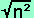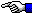# The Mathematical Error

Let's substitute some real numbers into the variables M and N. Let M=3 and N=5. So, (N-M) is 2, the average (A) is 4 and 2A is 8.

(N + M) (NM) = 2A (NM)     (1)
(5 + 3) x 2 = 8 x 2
16 = 16

N2 - M2 = 2AN-2AM    (2)
52 - 32 = (8 x 5) - (8 x 3)
25 - 9 = 40 - 24
16 = 16

N2 - 2AN = M2 - 2AM    (3)
25 - 40 = 9 - 24
-(16) = -(16)

N2 - 2AN + A2 = M2 - 2AM + A2    (4)
25 - 40 + 16 = 9 - 24 + 16
0 = 0

(N - A)2 = (M - A)2    (5)
(5 - 4)2 = (3 - 4)2
(1)2 = (-1)2
1 = 1

And in equation (6), taking the square root of both sides leads us to:

(N - A)2 = (M - A)2     --->     (N - A) = (M - A)     (6)

or:

(1)2  =  (-1)2     --->     ( 1 )  =  ( -1 )

Hold everything! You can't do that !  This is the error in the proof, and here's the reason why:

Since the square of a real number n (or n2) is never negative, then the square root of a real number squared () has meaning only if n is greater than or equal to zero. Without this rule, you would in effect be saying that the negative value of every number can be equal to its positive value! Obviously, we can't allow this sort of nonsense (that +1 = -1 ) to exist in such a logical system as Mathematics, so it is a fixed Rule of Mathematics that you must:

Never take the square root of a negative number.

( Note: This is similar to another Mathematical Rule that you must never divide by zero. )

# The Reality Error

Apart from this constant rule which we finally ran into, I'd also encourage anyone working with real-life applications of Mathematics to examine and state the conditions under which any particular problem must agree with before trying to solve it or provide a proof.

For example, although we finally ran into a mathematical rule showing this proof  to be incorrect, there was clearly a questionable operation performed prior to equation (6):  Although you might not think so, there's nothing wrong with using a negative number to represent the money here. A negative value would symbolize a debt or payment you might owe, thus it is possible to assign a negative value, for example, to cash you have borrowed or must have in the future to complete some project. However, most of the equations in this puzzle, such as equation (5), have a real problem: It would be similar to saying that a person can eliminate their debt to one creditor by simply multiplying it by yet another debt; and not only that, but gain the same amount of cash in the process! Sorry, but life doesn't work that way!! Our proof was still mathematically correct at that point, but it violated how you could apply that math to increasing personal wealth in real life. (Many people have been fooled into parting with their own money because they believed a scammer's lies that "seemed too good to be true!")

So, it's always a good idea when a problem deals with money (or more specifically, the lack of it), to state that we should never multiply two negative numbers together! [Accountants have often used red ink rather than a minus sign to symbolize debts versus credits for better understanding; we hate credit card bills, because they often use minus signs in very confusing ways!]

Whenever you're using mathematics to handle problems with physical objects, you must make sure that all the mathematical operations agree with reality for the present physical conditions of your objects!Back to The Starman's Math Index